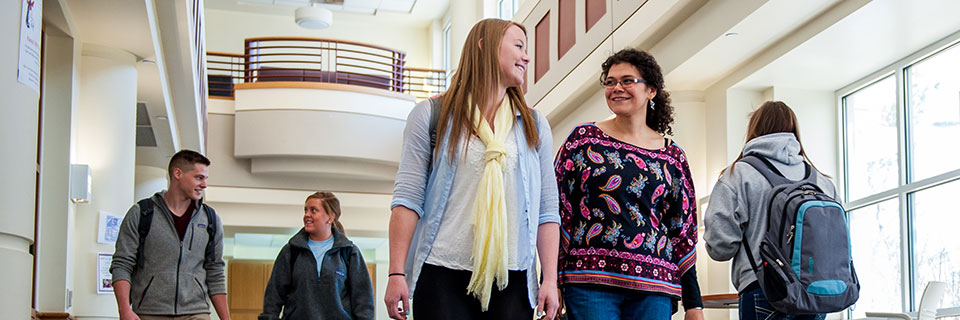# Mathematics Student Learning OutcomesGraduates with a mathematics major will:

• Use mathematical arguments to reason.
• Solve problems in novel settings using mathematics.
• Independently read mathematics in order to engage in mathematical reasoning.
• Effectively communicate mathematical ideas in writing.
• Effectively communicate mathematical ideas orally.
• Independently use the computer as a numerical, graphical, algebraic tool to aid their mathematical reasoning
• Complete the calculus 1, 2 and 3 sequence with a C or better.
• Complete an upper division course in linear algebra with a C or better.
• Complete one theoretical course with a C or better.